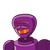# insert irrational between 2.324 and 2.412 please show the steps

insert irrational between 2.324 and 2.412

### 1 thought on “insert irrational between 2.324 and 2.412<br /> please show the steps”

1.### SOLUTION

TO DETERMINE

Insert irrational between 2.324 and 2.412

EVALUATION

We know that a Rational number is defined as a number of the form $$\displaystyle \sf{ \frac{p}{q} \: }$$

Where p & q are integers with $$q \ne \: 0$$

For example

$$\displaystyle \sf{2,- 1, \frac{1}{3} , – \frac{12}{23}} \: are \: the \: examples \: of \: rational \: numbers$$

Now a number which is not rational is called irrational number

Here the given numbers are 2.324 and 2.412

Hence a irrational between 2.324 and 2.412 is

= 2.37299378921878321.....

━━━━━━━━━━━━━━━━